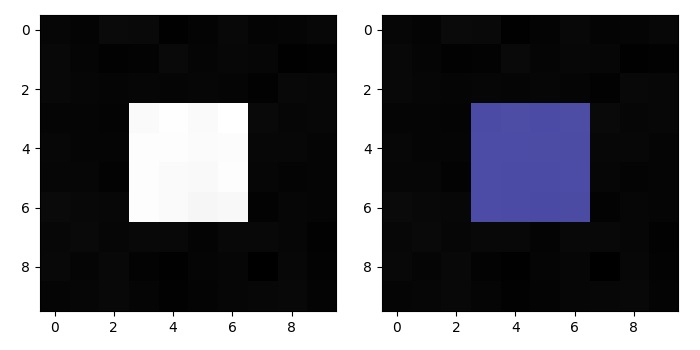# Overlay an image segmentation with Numpy and Matplotlib

To overlay an image segmentation with numpy, we can take the following Steps −

• Make a masked array of 10×10 dimension.

• Update the masked array with 1 for some region.

• Make image data using numpy.

• Mask an array where a condition is met, to get the masked data.

• Create a new figure or activate an existing figure using figure() mrthod.

• Use imshow() method to display data as an image, i.e., on a 2D regular raster.

• To display the figure, use show() method.

## Example

from matplotlib import pyplot as plt
import numpy as np
plt.rcParams["figure.figsize"] = [7.00, 3.50]
plt.rcParams["figure.autolayout"] = True
im = mask + np.random.randn(10, 10) * 0.01
plt.figure()
plt.subplot(1, 2, 1)
plt.imshow(im, 'gray', interpolation='none')
plt.subplot(1, 2, 2)
plt.imshow(im, 'gray', interpolation='none')
plt.show()

## OutputUpdated on: 15-May-2021

2K+ Views